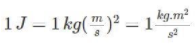• Call Now

1800-102-2727•

# Energy Definition, CGS Energy, MKS Energy, Energy Units List and Conversion of Energy

In simple words, energy can be described as the ability to perform work. It can take many different forms, including kinetic, potential, electrical, chemical, radioactive, thermal, and others. There are two types of energy: renewable and non-renewable. We'll also go through the different types of energy units in this article.

## What is the International System of Units (SI) for Energy?

The table of SI units is as follows:
SI Unit = Joules

Surprisingly, the name of this worldwide unit is preserved in commemoration of James Prescott Joule, a British physicist whose contributions to the development of the energy idea were important. When we look at the unit in its simplest form, 1-N.m equals 1 Joule, and in SI base units, it may be written as:## What is the CGS Energy Unit?

The unit table of the CGS system is as follows:
CGS Unit = erg
The erg is a unit of energy equal to 10⁻⁷ J. An erg is the amount of work performed by a single dyne over a one-centimeter distance.

You may also want to learn what are capacitors.

## What is the MKS Energy Unit?

The unit table of the MKS system is as follows:
MKS unit = Joule

## List of Energy Units

Energy units can be preceded by a variety of elements, and different units are typically used to measure energy. The following are some examples of common units:

1. British thermal unit (BTU)
2. Horsepower
3. Kilowatt-hour (kWh)
4. Calorie
5. Electronvolts (eV)
6. Hartree (the atomic unit of energy)
7. Rydberg units
8. Barrel of oil

## Conversion of Energy

The following is a table with a list of energy conversions:

 Unit Joule Calorie BTU Kilowatt-hour Joule 00 0.2390 0.000948 2.77778E-07 Calorie 4.187 00 0.00397 1.16279E-06 BTU 1055 252 00 0.000293 Kilowatt-hour 3.6E6 8.6E5 3412 00

## Commercial Energy Unit

The commercial unit of energy is the kilowatt-hour.

The SI unit of energy becomes little when huge amounts of energy need to be expressed. As a result, the commercial unit is employed.

## FAQs – Frequently Asked Questions

### What's the connection between energy and power?

• Power and energy are related in that power has units of energy that are split by time.
• Watts is the unit of power.
• Joules are a unit of energy.
• 1 Joule/1 second Equals 1 Watt

### When the unit of force and length are both doubled, what happens to the unit of energy?

When the units of force and length are doubled, the unit of unit energy grows fourfold.

The following is the relationship between energy, force, and distance:

• Force multiplied by distance equals energy.
• N and m are the units of force and distance, respectively.
• As a result, E = N x m
• As a result, E = 2 N x 2 m
• As a result, E = 4Nm.

### What's the difference between a commercial and a SI energy unit?

The commercial unit of energy is the kilowatt-hour (kWh). The quantity of energy utilised by a device in one working hour at a constant rate of one kilowatt is defined as one kilowatt-hour.

The Joule is the SI unit of energy.

As a result, the following is the relationship between the commercial and SI units of energy:

1 kWh = 1kW x 1h = 1000W x 1h = 1000(J/s) x 3600 s = 3.6 x106 J

### How many Joules does one unit of electrical energy equal?

3.6 106 Joules equals one unit of electrical energy.

### When does a spring reach its peak potential energy?

When a spring is either drawn out or squeezed, it will have the most energy.

### How many erg does one joule equal?

One joule equals 10⁻⁷ ergs.

### Examples of potential energy should be given.

Potential energy is exemplified by the following examples:

• Potential energy of gravity
• Potential energy that is elastic

### Make a list of the various types of kinetic energy.

The different types of kinetic energy are as follows:

• Kinetic energy in translation
• Kinetic vibrational energy
• Kinetic energy of rotationTalk to our expert
Resend OTP Timer =
By submitting up, I agree to receive all the Whatsapp communication on my registered number and Aakash terms and conditions and privacy policy